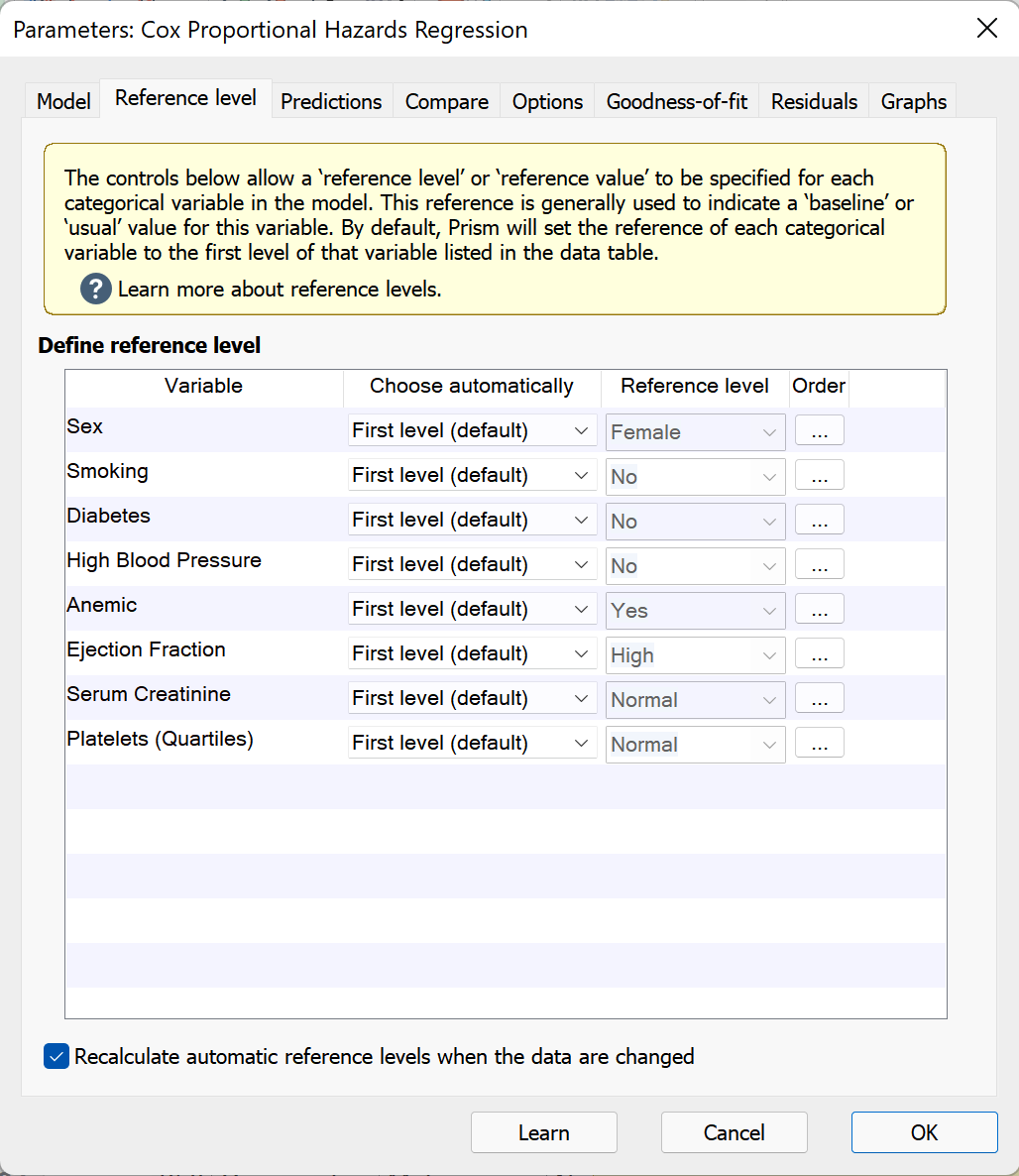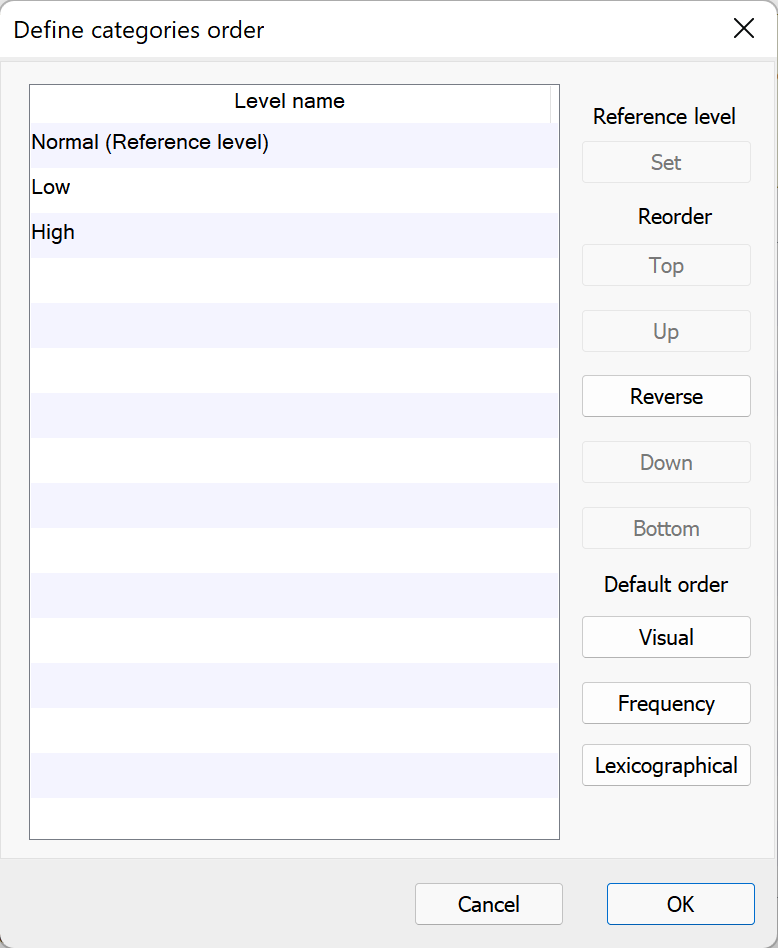## Please enable JavaScript to view this site.

 Setting reference levels for Cox regression

When a categorical predictor variable is included in a regression model as a predictor, Prism automatically encodes this variable using “dummy coding”. This process generates (behind the scenes) a number of new variables equal to the number of levels of the original categorical variable minus one. For example, if a categorical variable has five unique levels (A, B, C, D, and E), dummy coding would generate four new variables. If a categorical variable only has two unique levels (Male and Female, for example), dummy coding would only generate one variable. In this way, every level of a categorical predictor variable - except for one - gets a new variable that is used in the regression analysis. Additionally, a beta regression coefficient is calculated for each of these new variables.

But what do these beta coefficients represent? And what about the level that doesn’t get a new variable? These questions both have to do with the concept of a reference level for a categorical predictor variable.

## What are reference levels?

The reference level of a categorical predictor variable is often considered the “baseline” or “usual” value that is observed for the given variable. In the process of dummy coding, the variable for the reference level is left out since it would simply contain “0” for every observation. Instead, the reference level is used as a means of interpretation of the generated regression model. Let’s use an example to make this clear:

Consider a model that includes the categorical predictor variable “Sex” with levels “Male” and “Female”. If “Male” is our reference level, then the predicted model will include a beta coefficient (and hazard ratio) for “Female”, but will not include one for “Male”. The hazard ratio for “Female” in this case tells us is how many times greater or smaller the hazard rate is for women than for men, holding all other variables constant. In other words, if the hazard ratio for “Female” is 3.658, then the hazard rate expected to be 3.658 times greater for females than for males. Note that this interpretation can be applied directly to parameter estimates (beta coefficients) as well, but the idea of “log hazard” isn’t immediately intuitive, so we will often use hazard ratios (equal to exp(beta)) instead of beta directly when interpreting these values.## How to specify reference levels

On the Reference level tab, each of the categorical predictor variables included in the regression model will be listed under “Define reference level”. For each variable, you can choose to have Prism define the reference level automatically (based on a specified rule) or to define a level manually. When specifying a reference level automatically, there are a number of rules to choose from that Prism can use, including:

First level (default). Prism will select the first level of the variable in the data table. Note that if the order of the rows in the data table change, this reference level may also change!

Last level. Prism will select the final level of the variable in the data table. Note that if the order of the rows in the data table change, this reference level may also change!

Most frequent level. Prism will determine which level occurs most frequently within the variable, and use this as the reference level. This is good to use if you would like the regression coefficients to provide information on the effect of rare levels compared to common levels. Note that changing the order of the rows in the data table will not cause this reference level to change. However adding or removing data may cause the reference to change (by changing the frequency of each level)

Least frequent level. Prism will determine which level occurs least frequently within the variable, and use this as the reference level. Note that changing the order of the rows in the data table will not cause this reference level to change. However adding or removing data may cause the reference to change (by changing the frequency of each level)

For each of the rules above used to indicate how Prism should automatically select a reference level, certain changes to the data (organization or adding/removing data) may cause the automatically selected level to change. For example, if you’ve chosen the “Last level” rule for Prism to use, and add new rows of data to the bottom of the table, the “Last level” in the data table may change! Near the bottom of the Reference Level tab, there is a checkbox to “Recalculate automatic reference levels when the data are changed.” By default, this box is checked. However, if you would like to ensure that the reference levels do not change when the data are updated, you can uncheck this box. When unchecked, you can still use the rules to have Prism check for the corresponding level, but once you click “OK”, changes to the data will not cause any change to the specified reference levels (Prism will not “re-check” until you open the Reference Level tab and re-assign the rule).

Finally, you can also choose to specify a custom reference level by selecting “Custom…” in the first dropdown menu and selecting the desired level in the second dropdown menu.

## Changing the order of categorical variable levels in results

When generating the results output for regression analyses, Prism will display levels of categorical predictor variables in the same order that they appear in the data table. However, for the sake of presentation or publication, it may sometimes be useful to change the order of the levels for one or more specific categorical predictor variables in a regression model. The Order button in the “Define reference level” section allows you to customize the order of the levels of each categorical variable separately. Controls within the “Define categories order” submenu allow you to:

Set the reference level of the categorical variable to the currently selected level

Reorder the levels manually (Top, Up, Reverse, Down, and Bottom controls)

Reorder the levels using one of three default methods:

Visual order: the order that the levels first appear in the data table

Frequency: levels with greater frequency appear higher in the order

Lexicographical: the order is arranged using lexicographical order. Similar to alphabetical order, but note that a level named “a100” would be sorted before “a90” since “1” comes before “9”. This order does not consider the fact that the entire number “100” is greater than the entire number “90”## What happens to the reference levels if the input data changes?

By default, the reference level for a categorical variable is selected to be the first level of that variable in the data table. Prism also offers other automatic choices including "Last level", "Most frequent level", and "Least frequent level". However, if the input data are changed (or if additional data are added to the input data table), some of these automatic choices may also change. To ensure that a specified reference level does not change when the input data are changed or additional data is added, either uncheck the box beside "Recalculate automatic reference levels when the data is changed" or set the individual reference levels to "Custom..." using the appropriate dropdown menu.